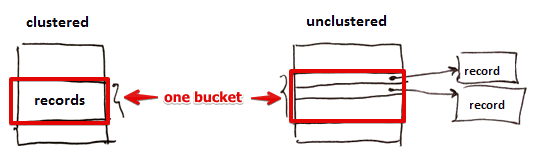# ML Wiki

## Motivation: Searching

Suppose we have a relation $R(A, B, C, D)$, each tuple - 32 bytes

• $128 \times 10^6$ tuples is $R$
• Block size is $B$ = 4kb
• I.e. can store 128 tuples per block, $10^6$ tuples in total

Suppose we want to find a tuple with $C = 10$

Searching in I/O Model of Computation

• for each block $X \in R$
• load $X$
• check if there's a tuple $T \in X$ with $C = 10$
• yes - output $T$
• no - continue
• release $X$ from memory

In worst case it's $10^6$ I/Os

• suppose $10^{-3}$ seconds per I/O operation
• 16.6 minutes in total

Can we do better?

## Indexing

An index is any secondary memory data structure that

• takes a search key as input
• efficiently returns the collection of matching records

## Clustered Index

Index can be clustered or unclustered

• When index is clustered it means the records themselves are stored in index, not pointers
• I.e. a clustered index ensures that all data is stored in some order
•• Usually there is only one clustered index per relation (otherwise the data will be duplicated)

If an index (say, B-Tree) is not clustered, then instead of following each pointer other techniques can be used, such as Bitmap Heap Scan

## Information Retrieval Indexing

Indexing can also be applied to unstructured data such as text